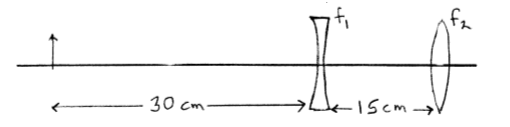# Problem: A small object that is 2 mm tall is placed 30 cm to the left of a diverging lens that has  f1 = -10cm. A converging lens with  f2 = +10 cm is 15 cm to the right of the diverging lens. How far is the final image from the converging lens?

###### Problem Details

A small object that is 2 mm tall is placed 30 cm to the left of a diverging lens that has  f= -10cm. A converging lens with  f= +10 cm is 15 cm to the right of the diverging lens. How far is the final image from the converging lens?